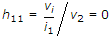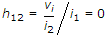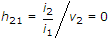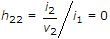# Electronics and Communication Engineering - Electronic Devices and Circuits

36.

The equivalent circuit of an ideal diode is

 A. a charging condenser B. a discharging condenser C. a switch D. a resistor

Answer: Option C

Explanation:

No answer description available for this question. Let us discuss.

37.

What is meant by "continuous collector current" in BJT?

 A. Maximum collector current B. Current at Quiescent condition C. Leakage current D. Junction Capacitance charging and discharging current

Answer: Option A

Explanation:

No answer description available for this question. Let us discuss.

38.

The small signal input impedance of a transistor whose output is shorted for the measuring signal is

 A.B.C.D.Answer: Option A

Explanation:

No answer description available for this question. Let us discuss.

39.

Assertion (A): The frequency of light used for photoelectric emission is high.

Reason (R): As per Einstein's equation 0.5 mv2 < hf - Uw.

 A. Both A and R are true and R is correct explanation of A B. Both A and R are true but R is not a correct explanation of A C. A is true but R is false D. A is false but R is true

Answer: Option A

Explanation:

No answer description available for this question. Let us discuss.

40.

The electric breakdown strength is affected by

 A. shape of the waveform of applied voltage B. steepness of the wavefront of the applied voltage C. composition of the material D. all of the above

Answer: Option D

Explanation:

No answer description available for this question. Let us discuss.

#### Current Affairs 2021

Interview Questions and Answers